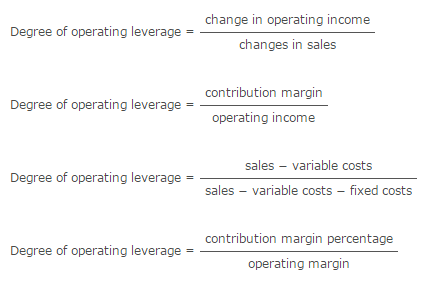Accounting

# Degree of Operating Leverage Formula

Degree of operating leverage is a measure of the extent of operating leverage i.e. the relationship between operating income and sales of a business. If operating income is more sensitive to changes in sales, the business is said to have high operating leverage and vice versa. Similarly, if operating profit margin is higher, the business is said to have high operating leverage and vice versa.

Formulas

Degree of operating leverage can be calculated using any of the following formulas:Examples

Calculate degree of operating leverage in the following cases and predict the increase in operating income subject to 15% increase in sales.

Company A: operating income increases by 15% if sales increase by 10%.

Company B: sales are \$2,000,000, contribution margin ratio is 40% and fixed costs are \$400,000

Solution

Company A:
Degree of operating leverage = % change in operating income/% change in sales = 15%/10% = 1.5
In response to a 15% increase sales, operating income will increase by 22.5% [=1.5 × 15%]

Company B:
Operating margin = (\$2,000,000 × 0.4 − \$400,000) ÷ \$2,000,000 = 20%
Degree of operating leverage = contribution margin percentage/operating margin = \$40% ÷ 20% = 2%

Increase in operating income in response to 15% increase in sales = 2 × 15% = 30%